## ­­From Y=X to Building a Complete Artificial Neural Network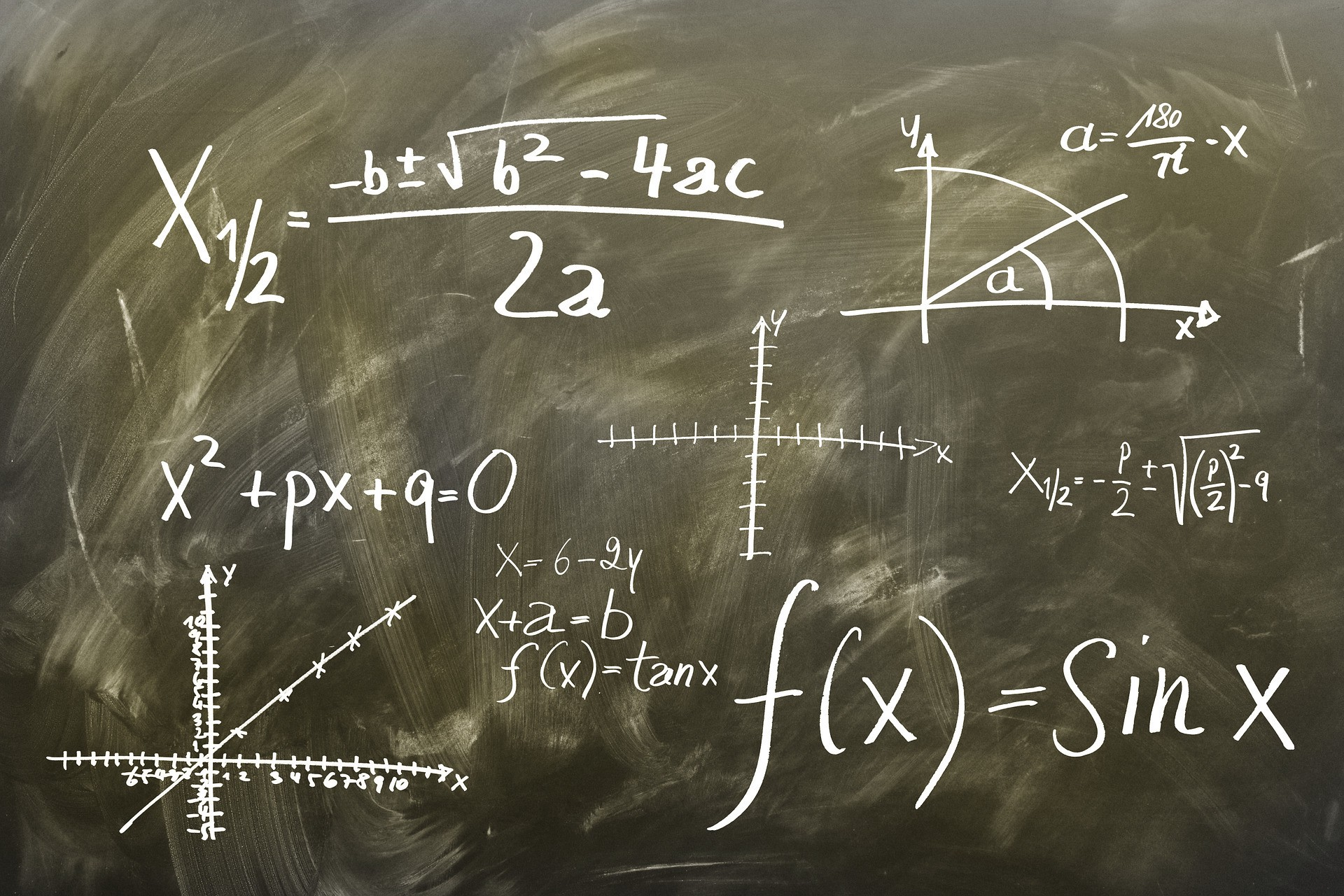At some point, you might have asked yourself, What are the origins of parameters for artificial neural networks? What is the purpose of the weight? What if no bias is used?

In this tutorial, we set out to answer those questions by working from the most simple artificial neural network (ANN), to something much more complex. Let’s start by building a machine learning model with no parameters—which is `Y=X`.

Then, we’ll gradually add some parameters to the model until we build a single neuron. This neuron is made to accept one or more inputs. The mathematical representation of the neuron is then mapped to a graphical representation. By connecting multiple neurons, a complete ANN can be created. After reading this tutorial, I hope that the purpose of the weights and bias are clear.### Starting by the Simplest Model Y=X

The building blocks of machine learning are actually quite simple. Even absolute beginners can build a basic machine learning model. Talking about supervised machine learning, its goal is to find (i.e. learn) a function that maps between the set of inputs and the set of outputs. After the learning itself is complete, the function should return the correct outputs for each given inputs. Let’s discuss one of the simplest tasks according to the data given in the next table.

There are 4 samples. Each sample has a single input and also a single output. After having a look at the data, we’ll need to prepare a function that returns the correct output for each given input with the least possible error. After looking at the data, we obviously notice that the output Y is identical to the input X. If X equals 2, then Y also equals 2. If X is 4, Y also is 4.

So what we need is a function that accepts a single input X and returns a single output. This output is identical to the input. With no doubt, the function is `F(X)=X`. For simplicity, we can replace F(X) by Y. So, the function will be `Y=X`.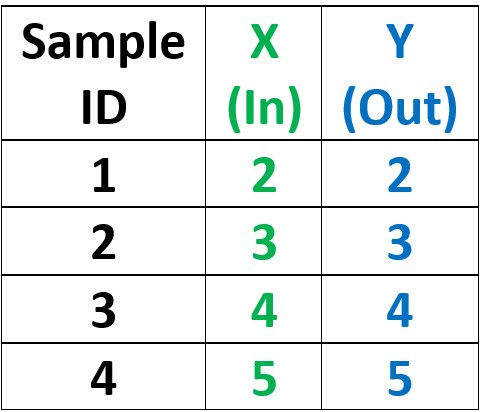### Error Calculation

After finding the suitable machine learning model (i.e. function), we need to test it to find whether it predicts the outputs correctly or if there is an error. We can use a simple error function that calculates the absolute difference between the correct output and the predicted output according to the next equation. It loops through the data samples, calculates the absolute difference between the correct and the predicted outputs for each sample, and finally sums all absolute differences and returns it into the error variable. The symbol N used in the summation operator represents the number of samples.The details of the calculations are given in the next table. According to that table, the function predicted all outputs correctly, and thus the total error is 0. GREAT. But do not forget that we’re working with one of the simplest problems for absolute beginners. Before changing the problem to make it a bit harder, I need to ask a question. In every machine learning model, there are 2 main steps which are learning (i.e. training) and testing. We’ve seen a basic testing step. But where is the learning step? Did we do learning in the previous model? The answer is NO.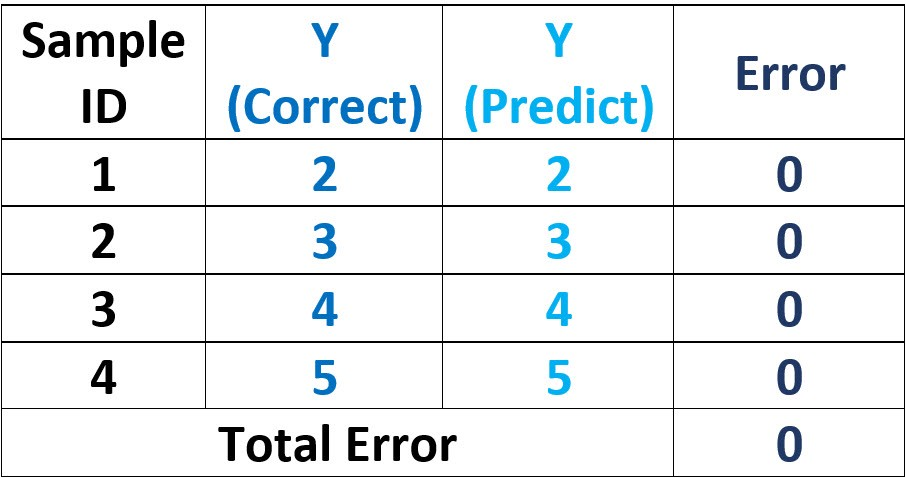Learning means that there are some parameters within the model that are learned from the data within the training step. The function of the previous model (`Y=X`) has no parameter to learn. The function just equalizes the input X and the output Y with no intermediate parameter to balance the relationship. In this case, there is no learning step because the model is non-parametric. Non-parametric means that the model has no parameters to learn from the data. The popular example of the non-parametric machine learning models is the K-nearest neighbors (KNN).

### Weight as a Constant

After clarifying that there is no parameter to learn, we can make a simple modification to the data used. The new data is given in the next table. Did you catch the modifications? It’s actually quite simple. Each output Y is no longer equal to the input X—it’s now double the input, which is 2X. We can still use the previous function (`Y=X`) to predict the outputs and calculate the total error.The details of the error calculations are given in the next table. The total error, in this case, is not 0 as in the previous example, but its value is 14. The existence of error within the data means that the model function cannot do the mapping between the input and the output correctly.

In order to reduce the error, we have to make some modifications over that function. The question is, what are the sources of modifications within this function (`Y=X`) that can reduce the prediction error? The function just has 2 variables X and Y. One represents the input and the other, the output. We cannot change either of them. As a conclusion, the function is non-parametric, so there’s no way to change it in order to reduce the error.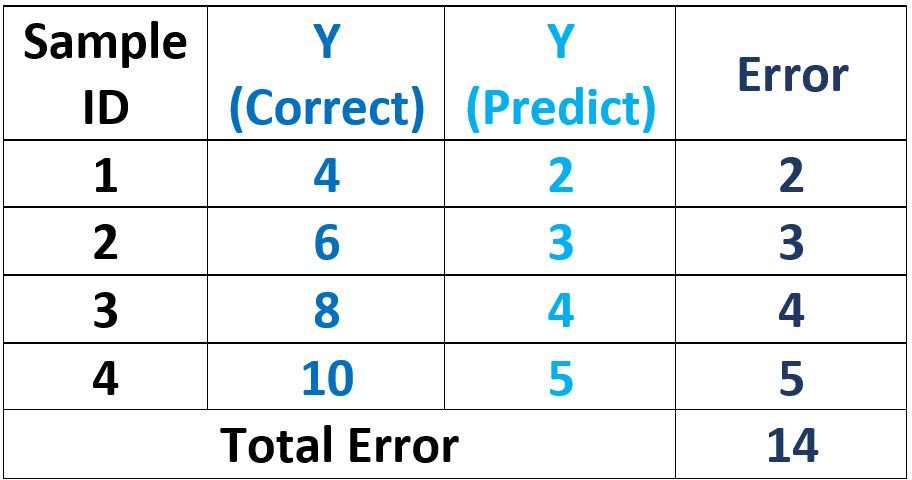But all is not lost! If the function currently doesn’t have a parameter, why not add one or more parameters? Don’t hesitate to design your machine learning model in ways that reduce the error. If you find that adding something to the function fixes the problem, start adding it at once.

In the new data, the output Y is double the input X. But the function isn’t changed to reflect this, and we still use `Y=X`. We can change the function by making the output Y equals 2X rather than X. The next function will be `Y=2X`. After using this function, the total prediction error is calculated according to the next table. The total error is now 0 again. NICE.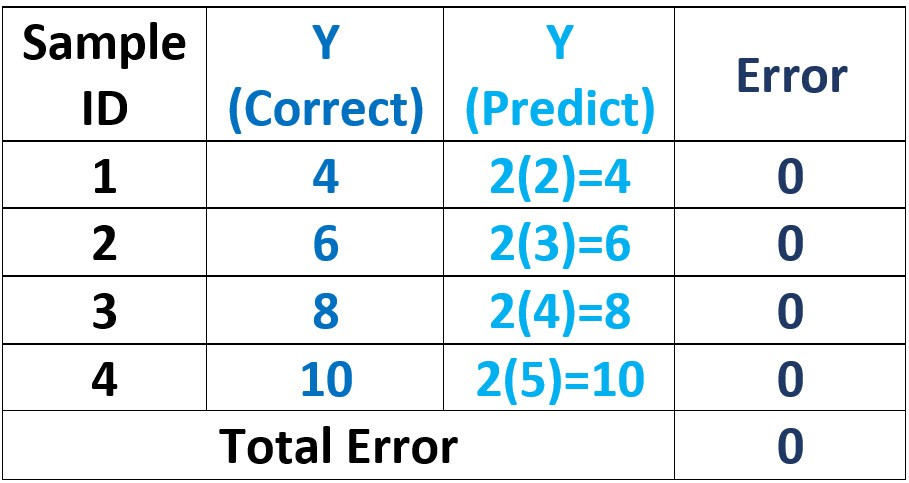After adding 2 to the function, does our model become parametric? NO. The model is still non-parametric. A parametric model learns the values of some parameters based on the data. Here, the value is calculated independently on the data, so the model is still non-parametric. The previous model has 2 multiplied by X but the value 2 is independent of the data. As a result, the model is still non-parametric.

Let’s change the previous data according to the next table.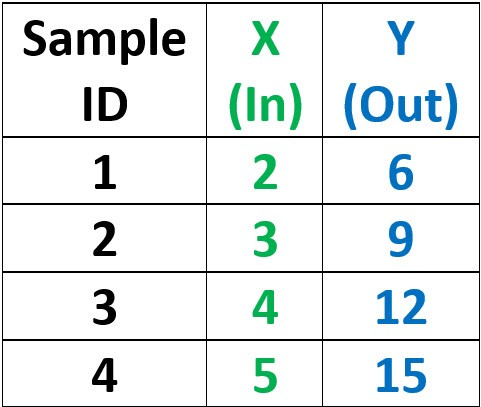Because there is no learning step, we can go ahead towards the testing step that calculates the prediction error after calculating the predicted output based on the last function (`Y=2X`). The total error is calculated according to the next table. The total error is no longer 0 but it is now 14. Why did that happen?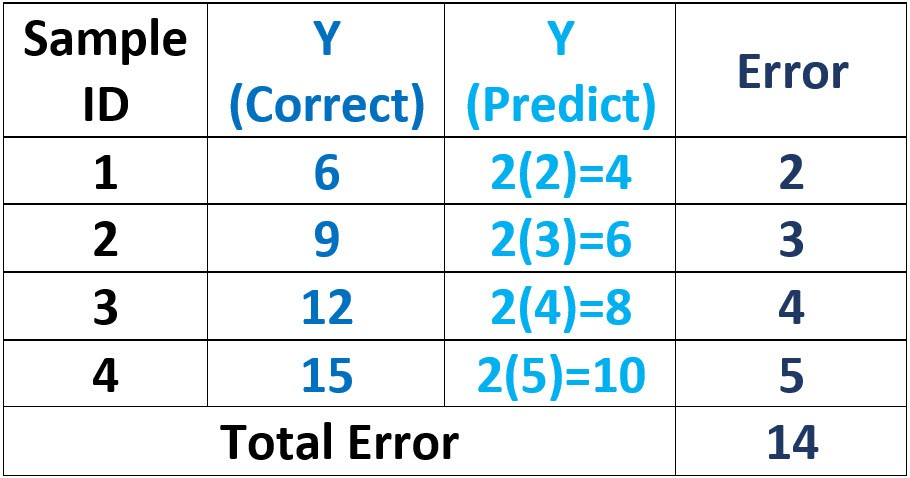The model used for solving this problem was created when the output Y is double the input (2X). Now, the output Y is no longer equal to 2X but 3X. So, it’s expected that we find an increase in the error. In order to eliminate this error, we have to modify the model function by using 3 rather than 2. The new function will be `Y=3X`.

The new function `Y=3X` will make the total error for the new data 0. But when working with the previous data in which Y is just double X, there will be an error. So, working with the proceeding data, we have to use 3 for getting multiplied by X to return a total error of 0. Working with the previous data, we had to change it to 2.

It seems that we have to change the model ourselves each time the data is changed. It’s tiresome. But there is a solution. We can avoid using constants in the function and replace them with variables. This is algebra—the field of using variables rather than constants.

### Weight as a Variable

Rather than using 2 in `Y=2X` or 3 in `Y=3X`, we can use a variable w in `Y=wX`. The value of the variable w is calculated based on the data. Because the model now includes a variable that has its value calculated based on the data, the model is now parametric. Because the model is parametric, there will now be a learning step within which the value of this variable (parameter) is calculated. This parameter is the weight of a neuron in the ANN. Let’s see how the model learns the value 2 of the parameter w when using the previous data in which Y equals to 2X. The data is given again below.

The process works by initializing the parameter w to an initial value that’s usually selected randomly. For each parameter value, the total error is calculated. Based on some values of the parameter, we can decide the direction in which the error reduces, which helps to select the best (optimal) value of the parameter.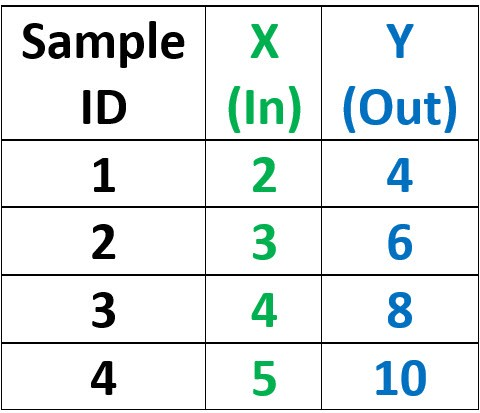### Optimizing the Parameters

Assuming that we selected an initial value of 1.5 for w, then our current function is `Y=1.5X`. We can calculate the total error based on this function according to the next table. The error is 8. Because there’s still an error, we can change the value of the parameter w.

But we don’t know the direction in which we should change the value of the parameter w. I mean, which is better? Should we increase or decrease the value of such a parameter? Because we don’t currently know, we can choose any value, either greater or smaller than the current value 1.5.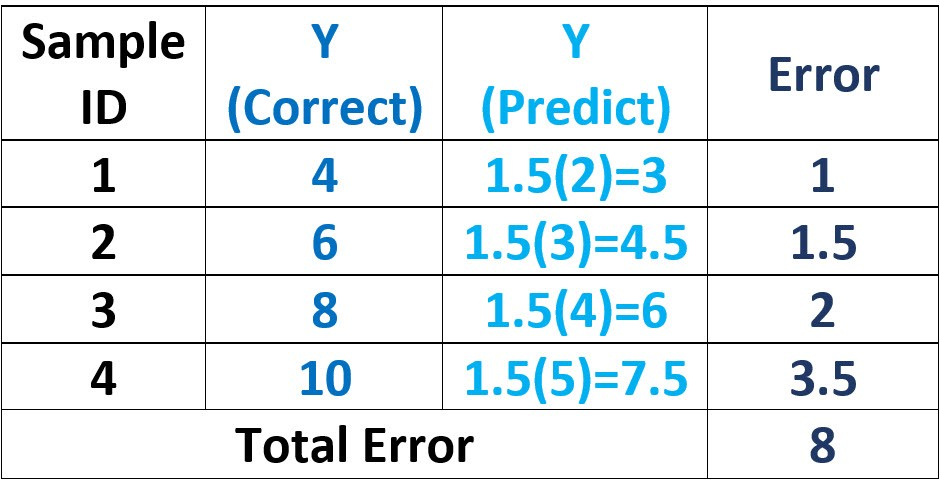Assuming that the new value of the parameter w is now 0.5, then the new function is `Y=0.5X`. We can calculate the total error based on this function. When `w=0.5`, the error is 21. Compared to the total error when we used the previous value of the parameter `w=1.5` which was 8, the error increased. This is an indication that we might be moving in the wrong direction. We can change the value of the parameter w to another value greater than 1.5 and see whether things are better.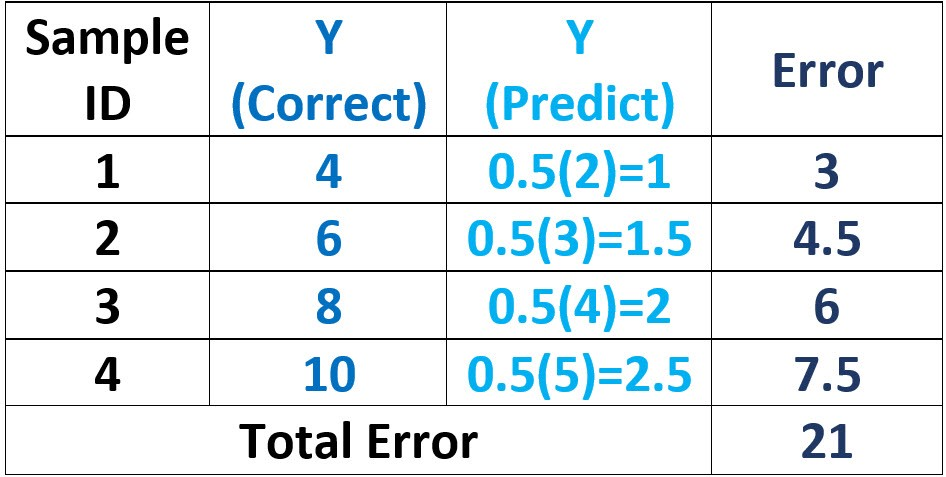If the new value is `w=2.5`, the new function will be `Y=2.5X`. Based on this function, the total error is now calculated according to the next table. The error is now 7, which is better than the previous 2 cases where the parameter w was equal to 1.5 and 0.5. So, when we increased the value of w than 1.5, the error reduced. We can continue increasing the value of w.Assuming that the new value for w is 3, the new function will be `Y=3X`. The total error is calculated based on this function according to the new table. The error is now 14. The error is now larger than before.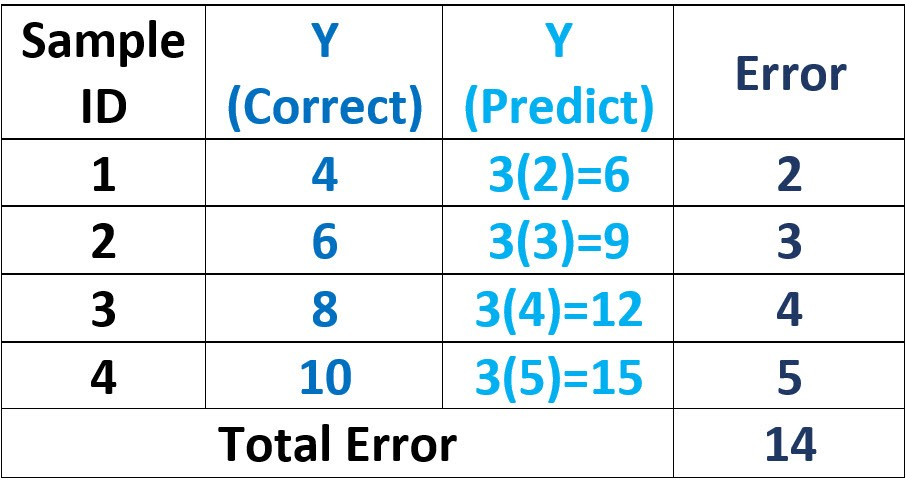To have a better view of the situation, we can summarize the previously selected values of the parameter w and their corresponding errors in the next table. The region of values for the parameter w that might reduce the error is bounded between 1.5 and 2.5. We can choose a value between such 2 values. The process will continue testing more values until finally concluding that the value of 2 is the best value that reaches the least possible error, which is 0. Finally, the function will be `Y=wX` when `w=2`.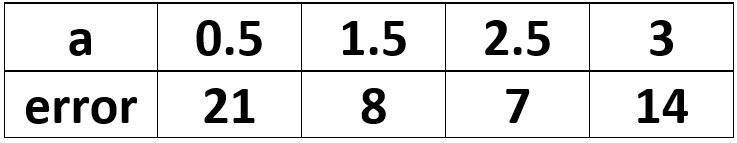This is for the data in which Y is equal to 2X. When the Y is equal to 3X, the process repeats itself until we find that the best value for the parameter w is 3. Up to this point, the purpose of using the weight in an ANN is now clear.

We can now discuss the purpose of the bias. To serve our purpose, we need to modify the data. The new data is given in the next table.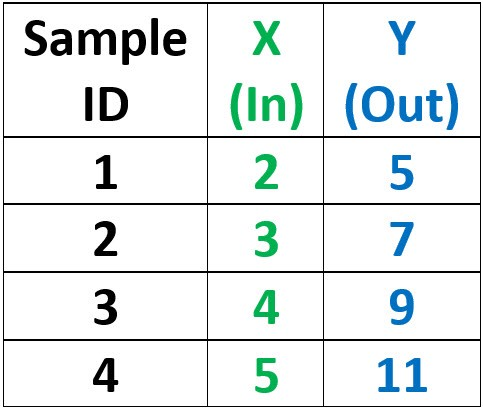### Bias as a Constant

This data is identical to the one used when `Y=2X`, but we’ve added a value 1 to each Y value. We can test the previous function `Y=wX` where `w=2` and calculate the total error according to the next table. There is a total error of 4.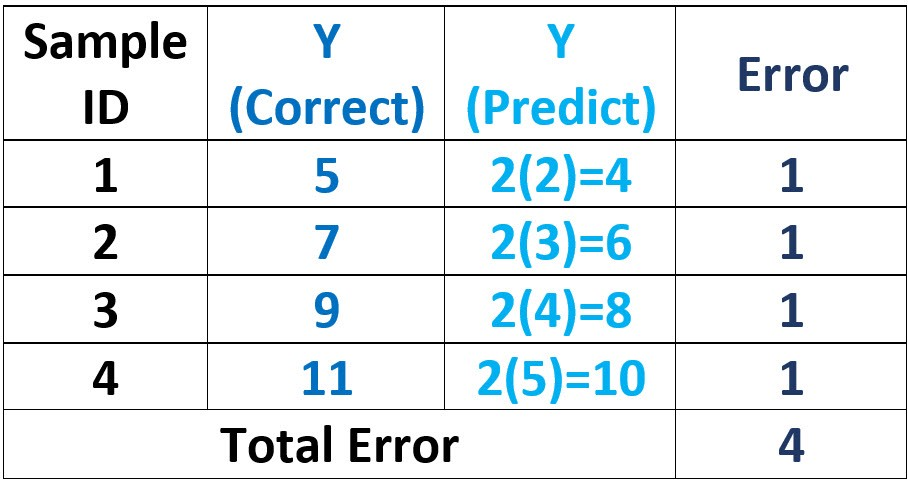According to our previous discussion, the error of means that the value of w is not the best and we have to change it until reaching an error of 0. But there are some cases in which using only the weights will not reach a 0 error. This example is a piece of evidence.

Using just the weight w, could we reach an error of 0? The answer is NO. Using just the weight in this example, we can approach the correct output, but there will be still an error. Let’s discuss this matter in a bit more detail.

For the first sample, what’s the best value for w in the equation `Y=wX` that returns an error equal to 0? It’s simple. We have an equation with 3 variables, but we know the values of 2 variables, which are Y and X. This leaves out a single variable w, which can be calculated easily using `w=Y/X`. For the first sample, Y equals 5 and X equals 2, and thus `w=Y/X=5/2=2.5`. So the optimal value for w that predicts the output of the first sample correctly is 2.5. We can repeat the same for the second sample.

For the second sample, `Y=7` and `X=3`. Thus, `w=Y/X=7/3=2.33`. So, the optimal value for w that predicts the output of the second sample correctly is 2.33. This value is different from the optimal value of w that works with the first sample. According to the 2 values of w for the first and second samples, we cannot find a single value for w that predicts their outputs correctly. Using `w=2.5` will leave an error in the second sample, and using `w=2.33` will leave an error for the first sample.

As a conclusion, using just the weight, we cannot reach an error of 0. In order to fix this situation, we have to use a bias.

An error of 0 can be reached by adding a value of 1 to the result of multiplication between w and X. So, the new function is `Y=wX+1` where `w=2`. According to the next table, the total error is now 0. GREAT.### Bias as a Variable

We’re still using a constant value of 1 to be added to wX. According to our previous discussion, using a constant value within the function makes this value dependent on a specific problem and not generic.

As a result, rather than using a constant of 1, we can use a variable, say b. Thus, the new function is Y=wX+b. The variable (parameter) b represents the bias in an ANN. When solving a problem, we now have 2 parameters, w and b, to decide their best values. This makes the problem a bit harder. Rather than finding the best value for just the weight w, we are asked to optimize 2 parameters w (weight) and b (bias). This takes much more time than before.

In order to find the optimal values for the 2 parameters, a good way is to start by optimizing just a single parameter until reaching the least possible error. After making sure that the error is not reduced anymore by changing this parameter, we can start optimizing the next parameter.

Applying this strategy to the previous example when optimizing the parameter w, we will notice that a tiny deviation from `w=2` increases the error. This is an indication that the value of 2 is the best value for the parameter w and we can start optimizing the next parameter b.

### From Mathematical Form to Graphical Form of a Neuron

At this point, we deduced a function `Y=wX+b` with 2 parameters. The first one is w representing the weight, and the second one is b representing the bias. This function is the mathematical representation of a neuron in ANN that accepts a single input. The input is X with a weight equal to w. The neuron also has a bias b.

By multiplying the weight (w) by the input (X) and summing the result by the bias (b), the output is Y, which is the output of the neuron that’s regarded as the input to the other neurons connected to it. The neuron can also be represented as a graph that summarizes all of this information, as shown in to figure below.

In the figure, you can find the mappings between the parameters in the mathematical function and the neuron graph. There is a single notice. The bias is regarded as a weight to an input of value +1. This makes it easy to manipulate the bias as a regular input.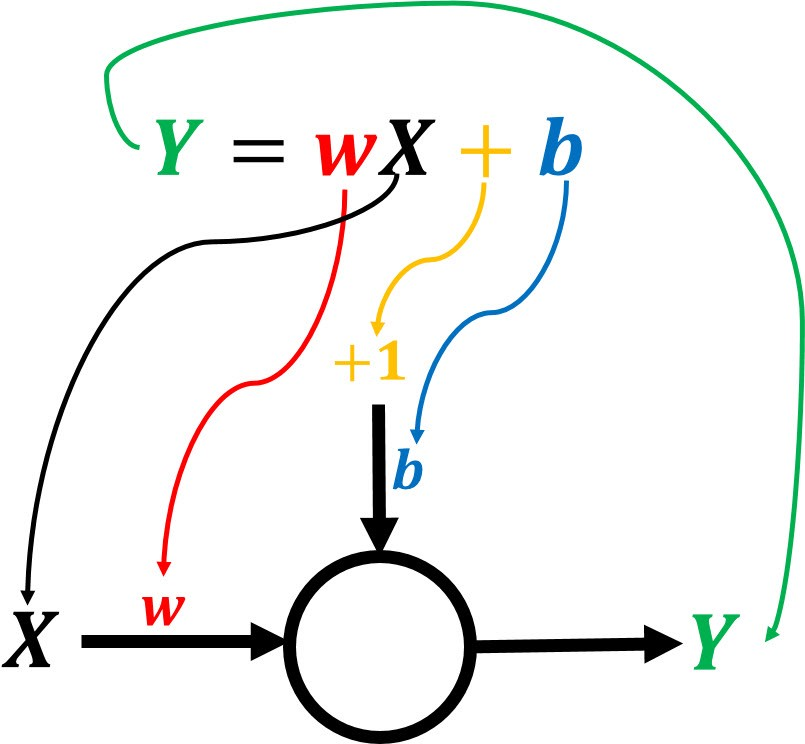### Neuron with Multiple Inputs

Up to this point, the purpose of the weight and the bias is now clear, and we’re also able to represent the neuron in both mathematical and graphical forms. But the neuron still accepts a single input. How do we allow it to support multiple inputs? This is also fairly simple. Just add whatever inputs you need in the equation and assign a weight to each of them. If there are 3 inputs, then the mathematical form will be as follows: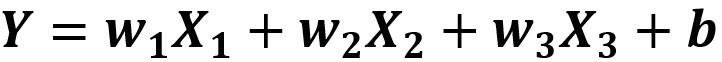Regarding the graphical form, just create a new connection for each input, then place the input and the weight on the connection. This is given in the next figure. By connecting multiple neurons of this form, we can create a complete artificial neural network. Remember that the starting point was just `Y=X`.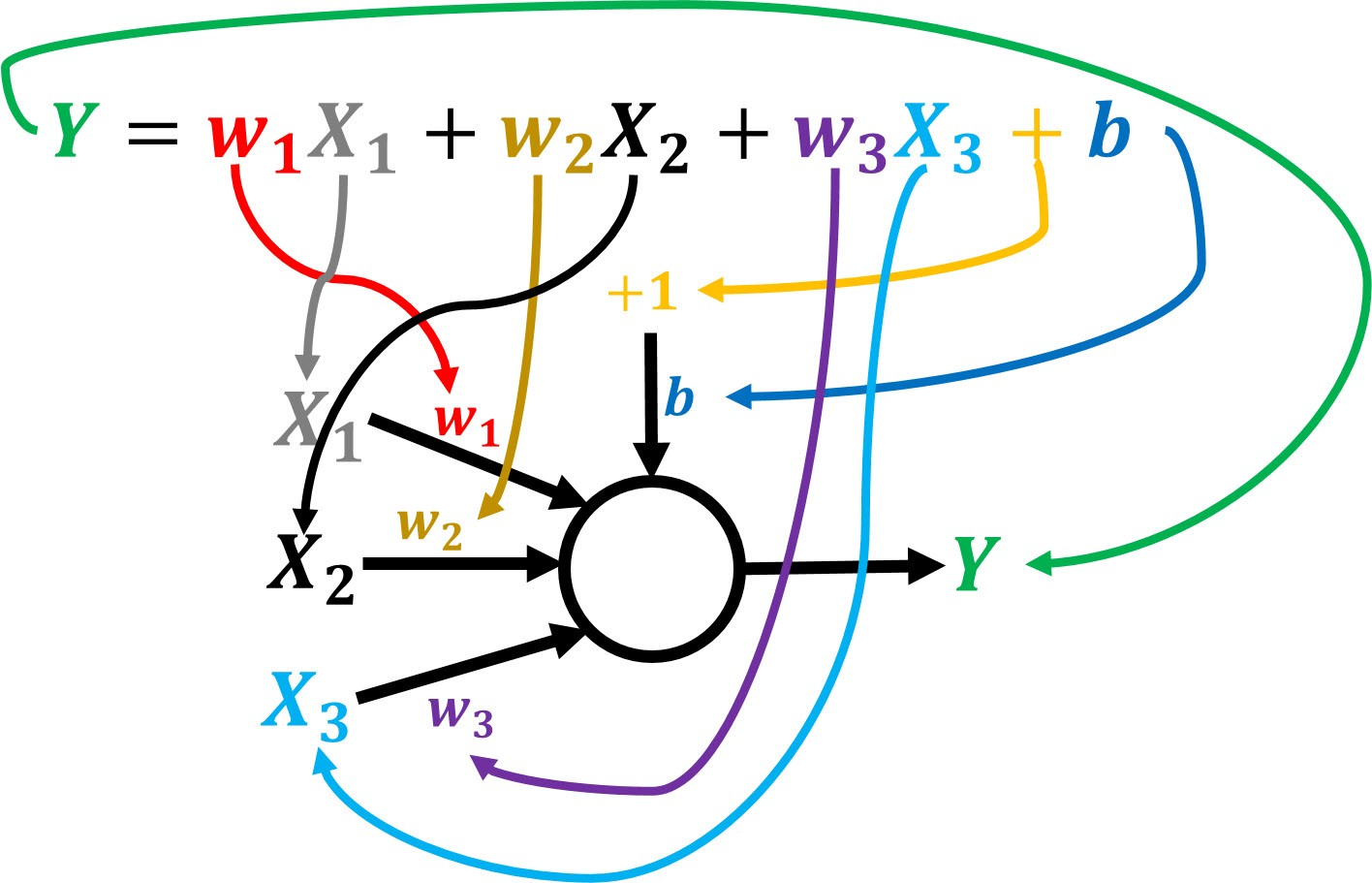### Sum of Products

In the mathematical form, we notice that different terms are repeated. These terms multiply each input by its corresponding weight. We can summarize all of these products within a summation operator. This operator will be responsible for returning the sum of products between each input and its corresponding weight.

The new mathematical form for the neuron is given below. Note that the summation starts from 0, not 1. This means there will be a weight (w) and an input (X) with indices 0. Such weight with index 0 will refer to the bias b. Its input will be always assigned +1.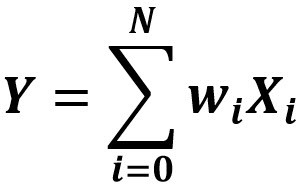You might also add the bias as a separate term added after the summation is completed (as shown below). In this case, the summation starts from 1.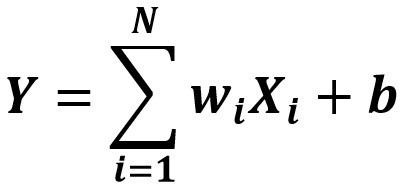### Conclusion

This tutorial provided a very detailed explanation of how to create a complete artificial neural network starting from a very simple function, `Y=X`. Throughout the tutorial, We explored the purpose of both weights and bias. Also, the tutorial mapped between the mathematical form and the graphical form of a neuron.

It is worth mentioning that this tutorial is based on my book Practical Computer Vision Applications Using Deep Learning with CNNs. So you can read more in this book, which is available through most popular distribution channels including Google Books, Amazon, Amazon Kindle, Springer, Apress, O’Reilly, and more. You can find the book at Springer at this link:

Practical Computer Vision Applications Using Deep Learning with CNNs
Deploy deep learning applications into production across multiple platforms. You will work on computer vision…

Bio: Ahmed Gad received his B.Sc. degree with excellent with honors in information technology from the Faculty of Computers and Information (FCI), Menoufia University, Egypt, in July 2015. For being ranked first in his faculty, he was recommended to work as a teaching assistant in one of the Egyptian institutes in 2015 and then in 2016 to work as a teaching assistant and a researcher in his faculty. His current research interests include deep learning, machine learning, artificial intelligence, digital signal processing, and computer vision.

Original. Reposted with permission.

Related: